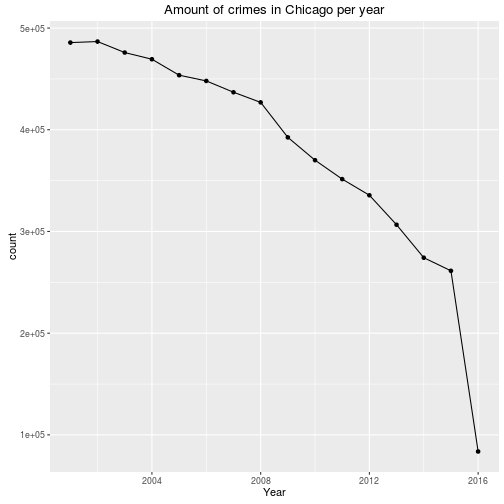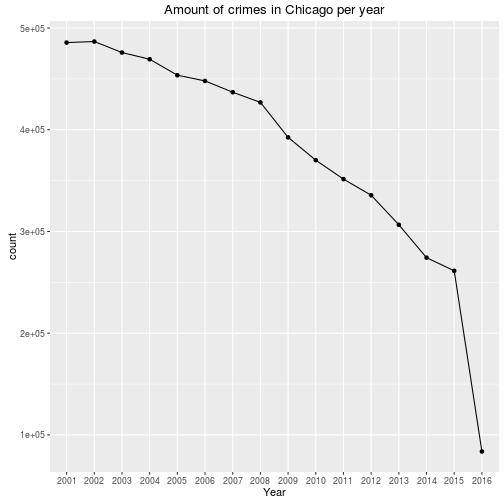# Use Spark and R to handle large files

Recently I saw a great webcast about the Spark connector for R. Since I am working with R and learning Apache Spark was already on my TODO-list, I tried it out.
Why would you use Spark in combination with R?
Well, R and RSstudio are great tools but as you probably know, it can't handle very large datasets very well. This is caused by the fact that R was designed to use only a single thread. Apache Spark (“Lightning fast cluster computing”), however is multi-threaded, so it can be more efficient to do your large file processing in Spark.
In this post, I will show to run Spark on your localhost, perform some action on a fairly large dataset (1.4GB) and use the result in R.

#### Setup SparkR

I have downloaded version 1.6.1 with pre-build for Hadoop 2.6 and later. SparkR can be run either from command line or from RStudio. Since I am mostly using RStudio, I will use the latter.

``````Sys.setenv(SPARK_HOME = "/home/ger/development/spark-1.6.1-bin-hadoop2.6")
Sys.setenv(SPARKR_SUBMIT_ARGS="--packages com.databricks:spark-csv_2.11:1.4.0 sparkr-shell")
.libPaths(c(file.path(Sys.getenv("SPARK_HOME"), "R","lib"),  .libPaths()))
library(SparkR)

#create context on localhost, use as many worker threads as logical cores on machine.
sc <- sparkR.init(master = "local[*]")
``````
```## Launching java with spark-submit command /home/ger/development/spark-1.6.1-bin-hadoop2.6/bin/spark-submit   --packages com.databricks:spark-csv_2.11:1.4.0 sparkr-shell /tmp/Rtmp6ro4R9/backend_portc8e9c0eb10
```
``````sqlContext <- sparkRSQL.init(sc)
``````

#### Handle a large file

I have chosen a dataset with a size, that is difficult to read directly in R, but should not be too big for memory. The dataset is about crimes in Chicago and can be downloaded from the city of chicago, it is about 1.4GB

First, I will read the data in the common way, via the read.csv function. Next, the data is grouped by year and plotted with ggplot.
The time it takes to perform these actions is measured.

``````library(sqldf)
library(ggplot2)
library(magrittr)

start.time <- Sys.time()
grouped_df <- sqldf("select Year, count(*) as count from df group by Year")
ggplot(data = grouped_df, aes(x= Year, y=count)) + geom_point() + geom_line(group=1) + ggtitle("Amount of crimes in Chicago per year")
````````````end.time <- Sys.time()
end.time - start.time
``````
```## Time difference of 4.092688 mins
```

SparkR

Now, let's create the same plot but use Spark for processing the data. Hopefully it will be quicker than plain R.
Below the code and time it takes to perform these actions with SparkR.

``````start.time <- Sys.time()
#read the data into a Spark DataFrame (capitals)
# add the data to a temp table to be able to use SQL
registerTempTable(DF, "crimes")
# define spark DataFrame with amount of crimes per year
groupedDF <- sqlContext %>% sql("select Year, count(*) as count from crimes group by Year")

#transfer to R. Since Spark is lazy, actual work is performed now!
grouped_df <- collect(groupedDF)
# stop spark
sparkR.stop()

#plot data in R
ggplot(data = grouped_df, aes(x= Year, y=count)) + geom_point() + geom_line(group=1) + ggtitle("Amount of crimes in Chicago per year")
````````````end.time <- Sys.time()
end.time - start.time
``````
```## Time difference of 23.12041 secs
```

#### Remarks

The time difference between plain R and SparkR is bigger that I thought! I know there are some other packages in R, that can also be used to parallelize and speed up the processing.
However, the nice thing with Spark is that it can also run on a cluster and thus scales well. Imagine, a dataset of 10TB, then the same approach can be used. Of course, in that case Spark should run on a cluster and not on my labtop:-)